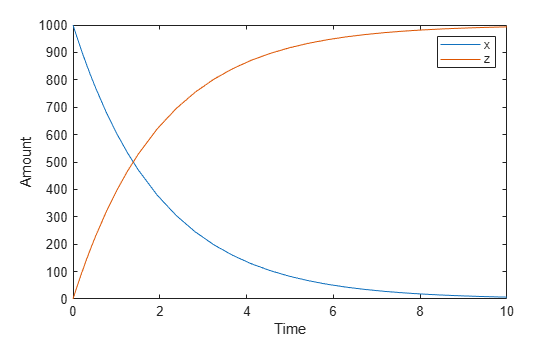# BoundaryCondition

Indicate species boundary condition

## Description

The `BoundaryCondition` property indicates whether reactions affect a species quantity.

When the `BoundaryCondition` of a species is `false` (default), the reactions can modify the species quantity. If a species is modified by reactions, then rules (repeated assignment rule, rate rule, or algebraic rule) cannot modify its value. SimBiology considers a reaction to modify a species when the net stoichiometry for the species is non-zero. For example, the reaction `X -> 2*X` modifies X, but the reaction ```X + E -> Y + E``` does not modify E.

When the `BoundaryCondition` of a species is `true`, then the value of the species is not changed by reactions during model simulation, even if the net stoichiometry is non-zero. Set the `BoundaryCondition` of a species to `true` if you want the species to participate in the reaction but you want to determine the value of that species using a rule instead. For example, set the `BoundaryCondition` of species X to `true` to specify its value using a repeated assignment rule but also to use species X in a reaction with `MassAction` kinetics, for example, ```X + Y -> Z```.

For details on how these two properties affect a species quantity during simulation, see How Species Amounts Change During Simulations.

Consider the following two use cases of boundary conditions:

• Modeling receptor-ligand interactions that affect the rate of change of the receptor but not the ligand. For example, in response to hormone, steroid receptors such as the glucocorticoid receptor (GR) translocate from the cytoplasm (`cyt`) to the nucleus (`nuc`). The hsp90/ hsp70 chaperone complex directs this nuclear translocation [Pratt 2004]. The natural ligand for GR is cortisol; the synthetic hormone dexamethasone (`dex`) is used in place of cortisol in experimental systems. In this system dexamethasone participates in the reaction but the quantity of dexamethasone in the cell is regulated using a rule. To simply model translocation of GR you could use the following reactions:

Formation of the chaperone-receptor complex,

```Hsp90_complex + GR_cyt -> Hsp90_complex:GR_cyt ```

In response to the synthetic hormone dexamethasone (`dex`), GR moves from the cytoplasm to the nucleus.

```Hsp90_complex:GR_cyt + dex -> Hsp90_complex + GR_nuc + dex ```
For `dex`,
` BoundaryCondition = true; ConstantAmount = false`
In this example `dex` is modeled as a boundary condition with a rule to regulate the rate of change of `dex` in the system. Here, the quantity of `dex` is not determined by the rate of the second reaction but by a rate rule such as

`ddex/dt = 0.001 `
which is specified in the SimBiology® software as
`dex = 0.001`

• Modeling the role of nucleotides (for example, GTP, ATP, cAMP) and cofactors (for example, Ca++, NAD+, coenzyme A). Consider the role of GTP in the activation of Ras by receptor tyrosine kinases.

`Ras-GDP + GTP -> Ras-GTP + GDP`
`For GTP, BoundaryCondition = true; ConstantAmount = true`

Model GTP and GDP with boundary conditions, thus making them boundary species. In addition, you can set the `ConstantAmount` property of these species to `true` to indicate that their quantity does not vary during a simulation.

## Characteristics

 Applies to Object: species Data type `boolean` Data values `true` or `false`. The default value is `false`. Access Read/write

## Examples

expand all

This example illustrates how to use the BoundaryCondition property of a species so that the species amount is not modified by the reaction it participates in, but by a user-defined dose object.

`sbioloadproject radiodecay.sbproj`

A SimBiology model named m1 is loaded to the MATLAB Workspace. The model is a simple radioactive decay model in which two species (x and z) are modified by the following reaction.k

`m1.Reactions`
```ans = SimBiology Reaction Array Index: Reaction: 1 x -> z ```

Simulate the model and view results before adding any boundary conditions.

```[t,x,names] = sbiosimulate(m1); plot(t,x); legend(names) xlabel('Time'); ylabel('Amount');```Add a RepeatDose object to the model and specify the species to be dosed, dose amount, dose schedule, and units.

```d1 = adddose(m1,'d1','repeat'); set(d1,'TargetName','z','Amount',100.0,'Interval',1.0,'RepeatCount',8); set(d1,'TimeUnits','second','AmountUnits','molecule');```

Set the BoundaryCondition of species z to be true so that the species will be modified by the dose object d1, but not by the reaction.

`set(m1.species(2),'BoundaryCondition',true);`

Simulate the model by applying the dose object.

`[t2,x2,names] = sbiosimulate(m1,d1);`

Plot the results. Notice that the amount of species z is now modified by the repeated dose object, but not by the reaction.

```[t2,x2,names] = sbiosimulate(m1,d1); plot(t2,x2); legend(names); xlabel('Time'); ylabel('Amount');```## References

Pratt, W.B., Galigniana, M.D., Morishima, Y., Murphy, P.J. (2004), Role of molecular chaperones in steroid receptor action, Essays Biochem, 40:41-58.